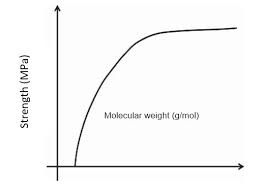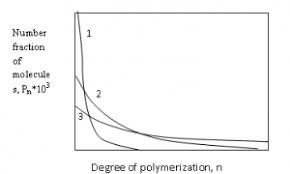## How to Calculate and Solve for Tensile Strength of Polymers | Polymer

The tensile strength of polymers is represented by the image below.To compute for tensile strength of polymers, three essential parameters are needed and these parameters are Tensile Strength at Infinite Weight (TS), Constant (A) and Mean Number Molecular Weight (M’n).

The formula for tensile strength of polymers:

TS = TS – (A/M’n)

Where:

TS = Tensile Strength of Polymers
TS = Tensile Strength at Infinite Weight
A = Constant
M’n = Mean Number Molecular Weight

Let’s solve an example;
Find the tensile strength of polymers when the tensile strength at infinite weight is 8, the constant is 4 and the mean number molecular weight is 2.

This implies that;

TS = Tensile Strength at Infinite Weight = 8
A = Constant = 4
M’n = Mean Number Molecular Weight = 2

TS = (8) – (4/2)
TS = (8) – (2)
TS = 6

Therefore, the tensile strength of polymers is 6 Pa.

Calculating the Tensile Strength at Infinite Weight when the Tensile Strength of Polymers, Constant and the Mean Number Molecular Weight is Given.

TS = TS – (A / M’n)

Where:

TS = Tensile Strength at Infinite Weight
TS = Tensile Strength of Polymers
A = Constant
M’n = Mean Number Molecular Weight

Given an example;
Find the tensile strength at infinite weight when the tensile strength of polymers is 44, the constant is 80 and the mean number molecular weight is 10.

This implies that;

TS = Tensile Strength of Polymers = 44
A = Constant = 80
M’n = Mean Number Molecular Weight = 10

TS = TS – (A / M’n)
TS = 44 – (80 / 10)
TS = 44 – 8
TS = 36

Therefore, the tensile strength at infinite weight is 36.

## How to Calculate and Solve for Degree of Polymerization | PolymerThe image of degree of polymerization is represented above.

To compute for degree of polymerization, two essential parameters are needed and these parameters are Mean Number Molecular Weight (M’nand Unit Molecular Weight (m).

The formula for calculating degree of polymerization:

D.P. = M’n/m

Where:

D.P. = Degree of Polymerization
M’n = Mean Number Molecular Weight
m = Unit Molecular Weight

Let’s solve an example;
Find the degree of polymerization when the mean number molecular weight is 28 and the unit molecular weight is 4.

This implies that;

M’n = Mean Number Molecular Weight = 28
m = Unit Molecular Weight = 4

D.P. = 28/4
D.P. = 7

Therefore, the degree of polymerization is 7.

Calculating the Mean Number Molecular Weight when the Degree of Polymerization and the Unit Molecular Weight is Given.

M’n = D.P (m)

Where;

M’n = Mean Number Molecular Weight
D.P. = Degree of Polymerization
m = Unit Molecular Weight

Let’s solve an example;
Find the mean number molecular weight when the degree of polymerization is 6 and the unit molecular weight is 4.

This implies that;

D.P. = Degree of Polymerization = 6
m = Unit Molecular Weight = 4

M’n = D.P (m)
M’n = 6 (4)
M’n = 24

Therefore, the degree of polymerization is 24.